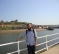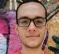## correlation for complex numberStarted by 5 years ago12 replieslatest reply 5 years ago9495 views

Hi,

This question may be simple by I was surprised that it is not easy to find an answer by asking around or googling :

I am wondering why when we calculate correlation of 2 complex number we always use the conjugate on one of them. Are we sort of trying to get rid of the imaginary part here or something like that?

Thanks!

[ - ]Hello,

@jtrantow gave a very good explanation. I will try to summarize it according to my understanding.

The correlation is a measure of similarity between signals (vectors). If we interpret signals as vectors in the N-dimensional space, the correlation becomes simply the projection of the two vectors, as @jtrantow stated. In the case, the angle between the vectors is required. Here comes the conjugate role. Let us derive it mathematically:

Let x = a exp(j theta)

y = b exp(j phi)

Then, the correlation is defined as

x.conj(y) = a exp(j theta) . conj(b exp(j phi))

= a exp(j theta) . (b exp(-j phi)

= ab cos(theta - phi)

which makes sense, since we are interested in the angle between the vectors (theta - phi) not the absolute angles (theta, phi).

[ - ]There are multiple correlations possible for complex data.

What you roughly want for a correlation is a value that is 1 when the numbers are the same. -1 when the signals are opposite, and between -1 and 1 depending on how closely the signal are. A 0 value indicates numbers are not correlated.

If you visualize two complex numbers in Cartesian coordinates you can consider the geometric lengths and the angle between the two numbers. The dot/inner product is defined by these lengths and the angle between the two numbers. Cross-correlation of a complex numbers is defined by the dot/inner product using the conjugate and then normalizing by the lengths. You can also think of the dot/inner product as a projection.

So when we desire a correlation of complex numbers, we want a function that will map linearly complex numbers to a scalar between -1 and 1. Euclidean geometry figured this out long ago. Modern math classes cover this in Linear Algebra. Advanced math classes cover things like normed vector space, inner product spaces, Hilbert spaces. In the big picture, this progression takes the same ideas (length, angles) from 2D Euclidean dimensions and extend to finite and infinite dimensions.

Bottom line, there is nothing magical about the conjugate. Understand the geometrical concept and realize the conjugate is just a mathematical helper for calculating the angle and lengths.

P.S. Lets try to be specific.

Complex number: a+ib.

Complex function: f(t) = a(t) + jb(t).

[ - ]Lecture on projection

[ - ]48 minutes Video here!! can anybody confirm I am not hallucinating.

[ - ]Very good explanation!

[ - ]Your question is related to the definition of an inner product space over the field of complex numbers (see this). Such definition gives a nice, natural extension of the norm induced by the inner product we see in the real case (but this is like a start-from-the-end answer, I don't know the actual reason). Once expectation is a linear operator, you can also verify that <X,Y> = E(X * conj(Y)) defines an inner product for a space of random variables (usually with the constraint of having finite second moments). There is an additional technicality in the case of random variables related to the third axiom of the above link: we can't talk in a deterministic manner about random events so that the condition turns into "<X,X> = 0 <=> X = 0 with probability 1". I hope this helps.

Best,

Abel

[ - ]Because they are generally phasors.

a(t) = re(Ae^jwt)

b(t) = re(Be^jwt)

we calculate re(A*conj(B)) = re(conj(A)*B)) = re(Ae^jwt * conj(Be^jwt)) = re(A*conj(B)) since e^jwt * conj(e^jwt) = e^jwt * e^(-jwt) = 1

[ - ]Are you talking about correlation in frequency domain? if so the conjugate means reversal of stream (compared to time domain).

[ - ]Actually I came across calculating "noise covariance matrix" during some study where we take the same noise vector N and do sort of correlation to itself, and couldn't understand why we need to take N and conjugate transpose of N to calculate the covariance matrix R{nn^H} instead of just taking N and and N transpose, e.g. R{nn^T}

[ - ]The transpose is just a simplification of the complex conjugate that only applies to real numbers and matrices. You won't go wrong if you always use the complex conjugate/Hermitian.

[ - ]I think it is now clear how best to correlate two complex vectors. The horse has been killed.

I have been doing this sort of correlation for years in Matlab without realising that matlab function does actually conjugate one of the vectors. Here is the proof, I am comparing conjugation against no conjugation using reverse convolution:

%%%%%%%%%%%%%%%%%%%%%%%%%%%

x1 = randn(1,10)+j*randn(1,10);

x2 = x1; %randn(1,10)+j*randn(1,10);

%y = xcorr(x1,x2);       %matlab function includes conjugation

y1 = conv(x1,fliplr(x2)); %no conjugation

x2 = conj(x2);           %conjugation

y2 = conv(x1,fliplr(x2));

plot(abs(y1),'.-');hold;

plot(abs(y2),'r.--');

%%%%%%%%%%%%%%%%%%%%%%%%%%

What this thread also raises is the wording and somehow the failure of inner products concept.

We want to correlate A & B but then we actually correlate A with conjugated B

Are we saying that the sum of inner complex products fails here? I think it does.

Kaz

[ - ]Matlab's xcorr() returns the cross-correlation of two discrete-time sequences.